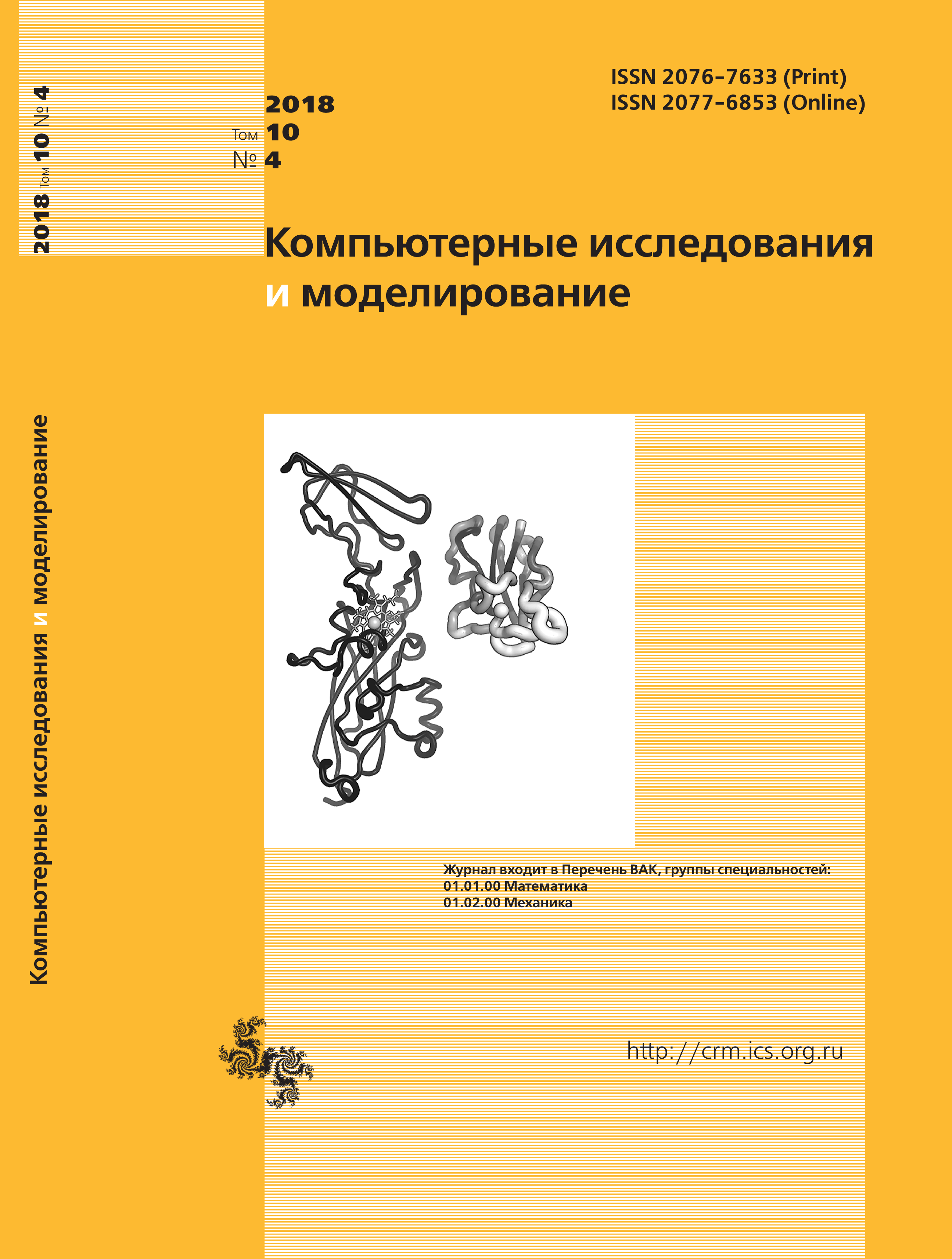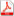Issue 4, 2018 Vol. 10

# All issues

Direct multiplicative methods for sparse matrices. Quadratic programmingpdf (322K)  / List of references

A numerically stable direct multiplicative method for solving systems of linear equations that takes into account the sparseness of matrices presented in a packed form is considered. The advantage of the method is the calculation of the Cholesky factors for a positive definite matrix of the system of equations and its solution within the framework of one procedure. And also in the possibility of minimizing the filling of the main rows of multipliers without losing the accuracy of the results, and no changes are made to the position of the next processed row of the matrix, which allows using static data storage formats. The solution of the system of linear equations by a direct multiplicative algorithm is, like the solution with LU-decomposition, just another scheme for implementing the Gaussian elimination method.

The calculation of the Cholesky factors for a positive definite matrix of the system and its solution underlies the construction of a new mathematical formulation of the unconditional problem of quadratic programming and a new form of specifying necessary and sufficient conditions for optimality that are quite simple and are used in this paper to construct a new mathematical formulation for the problem of quadratic programming on a polyhedral set of constraints, which is the problem of finding the minimum distance between the origin ordinate and polyhedral boundary by means of a set of constraints and linear algebra dimensional geometry.

To determine the distance, it is proposed to apply the known exact method based on solving systems of linear equations whose dimension is not higher than the number of variables of the objective function. The distances are determined by the construction of perpendiculars to the faces of a polyhedron of different dimensions. To reduce the number of faces examined, the proposed method involves a special order of sorting the faces. Only the faces containing the vertex closest to the point of the unconditional extremum and visible from this point are subject to investigation. In the case of the presence of several nearest equidistant vertices, we investigate a face containing all these vertices and faces of smaller dimension that have at least two common nearest vertices with the first face.

Keywords: mathematical programming, quadratic programming, sparse matrices, direct multiplicative algorithm, a new mathematical formulation, necessary and sufficient conditions of optimality, quadratic problem, linear programming, multi-dimensional geometry
Citation in English: Sviridenko A.B. Direct multiplicative methods for sparse matrices. Quadratic programming // Computer Research and Modeling, 2018, vol. 10, no. 4, pp. 407-420
Citation in English: Sviridenko A.B. Direct multiplicative methods for sparse matrices. Quadratic programming // Computer Research and Modeling, 2018, vol. 10, no. 4, pp. 407-420
DOI: 10.20537/2076-7633-2018-10-4-407-420
Views (last year): 32.

Full-text version of the journal is also available on the web site of the scientific electronic library eLIBRARY.RU

The journal is included in the Russian Science Citation Index

The journal is included in the List of Russian peer-reviewed journals publishing the main research results of PhD and doctoral dissertations.

International Interdisciplinary Conference "Mathematics. Computing. Education"

The journal is included in the RSCI

Indexed in Scopus